Students can access the CBSE Sample Papers for Class 11 Chemistry with Solutions and marking scheme Term 2 Set 1 will help students in understanding the difficulty level of the exam.

## CBSE Sample Papers for Class 11 Chemistry Standard Term 2 Set 1 with Solutions

Max. Marks: 40
Time: 2 Hours

General Instructions:

• There are 12 questions in this question paper with internal choice.
• Section A: Q. No. 1 to 3 are very short answer questions carrying 2 marks each.
• Section B: Q. No. 4 to 11 are short answer questions carrying 3 marks each.
• Section C: Q. No. 12 is case-based question carrying 5 marks.
• All questions are compulsory.
• Use of log tables and calculators is not allowed.

Section-A

Question 1.
Attempt any two:
(i) Define physical equilibrium. Give an example also.
(ii) Define chemical equilibrium. Give an example.
(iii) Why is chemical equilibrium called dynamic equilibrium?
(i) A physical equilibrium is a system whose physical state does not change when dynamic equilibrium is reached.
e.g., Solid ↔ liquid.
(ii) A chemical equilibrium is a system whose chemical composition of the system does not change when dynamic equilibrium is reached.
e.g., N2(g) + 3H2(g) ↔ 2NH3(g)

(iii) Chemical equilibrium is achieved when the rate of forward and backward reaction becomes equal. Although overall it is in equilibrium but inside the forward reaction and backward reaction both occur and so there is motion in the atomic and molecular level. Dynamic refers to anything having motion. Since the equilibrium is not static so, the chemical equilibrium is called dynamic equilibrium.Question 2.
What will be the minimum pressure required to compress 500 dm3 of air at 1 bar to 200 dm3 at 30°C?
Given,
P1= 1 bar
P2=?
V1 = 500dm3
V2 = 200dm3
Temperature remains constant at 30°C.

∴ According to Boyle’s law,
P1V1 = P2V2
1 x 500=P2 x 200
P2 = 2.5bar

Question 3.
(i) Alkali metals should be stored in kerosene oil.
(ii) Lithium is kept wrapped in paraffin wax and not stored in kerosene.
(i) The alkali metals tarnish in air due to the formation of oxide at their surface and hence they are stored in kerosene oil.2Na + O2 → Na2O2
M+O2 → MO2
[where, M = K, Rb, Cs]

(ii) Lithium cannot be stored in kerosene as it floats on the surface of kerosene because of its low density. Therefore, lithium is generally kept wrapped in the paraffin wax.Section B

Question 4.
Account for the following:
(i) Benzene undergo electrophilic substitution reactions easily but nucleophilic substitutions with difficulty.
(ii) Rotation around carbon-carbon single bond of ethane is not completely free.
(iii) Halogens are o- and p-directing in haloarenes despite their -I effect.
OR
How will the following conversions take place? Give chemical reaction of each of the following.
(i) Ethyne into benzene.
(ii) Propene to propane-l-ol.
(iii) Benzene to m-nitrochlorobenzene
(i) Benzene is a planar molecule having delocalized electrons above and below the plane of ring. Hence, it is electron-rich compound. As a result, it is highly attractive towards electron-deficient species i.e., electrophiles. Therefore, it undergoes electrophilic substitution reactions very easily. Nucleophiles are electron-rich in nature. Hence, they are repelled by nucleophilic character of benzene. Hence, benzene undergoes nucleophilic substitutions with difficulty.

(ii) Ethane contains a carbon-carbon sigma (σ) bond. Electron distribution of the sigma molecular orbital is symmetrical around the internuclear axis of the C-C bond which is not disturbed due to rotation about its axis. This permits free rotation around a C-C single bond. However, rotation around a C-C single bond is not completely free. It is hindered by a small energy barrier due to weak repulsive interaction between the adjacent bonds.

Such a type of repulsive interaction is called torsional strain. From all the conformations of ethane, the staggered form has the least torsional strain and the eclipsed form has the maximum torsional strain. The energy difference between the two extreme forms is of the order of, 12.5 kJ mol-1, which is very small. It has not been possible to separate and isolate different conformational isomers of ethane.

(iii) In case of aryl halides, halogens are little deactivating because of their strong -I effect. Therefore, overall electron density on the benzene ring decreases. In other words, halogens are deactivating due to -I effect. However, because of the + R-effect, i.e., participation of lone pairs of electrons on the halogen atom with the π-electrons of the benzene ring, the electron density increases more at o- and p-positions than at m-positions. As a result, halogens are o-, p-directing. The combined result of + R-effect and -I-effect of halogens is that halogens are deactivating but o, p-directing.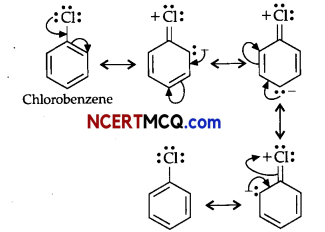OR
(i) Benzene from Ethyne: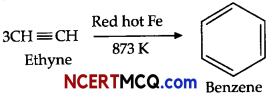(ii) Addition of water to propene in presence of few drops of sulphuric acid (catalyst) and peroxide leads to the formation of propan-1-ol.(iii)Benzene can be converted into m-nitrochlorobenzene as:Question 5.
(i) Under what conditions is the heat absorbed by a system is equal to the work done by the system?
(ii) Under what conditions the following reaction will be spontaneous:
(a) Reaction with both ΔH and ΔS positive.
(b) Reaction with both ΔH and ΔS negative.
OR
(i) What is enthalpy change at constant volume? Explain.
(ii) Calculate the enthalpy of transition for carbon from the following data given:
Cdiamond + O2 → CO2(g); ΔH = – 943 kcal
Camorphous + O2 → CO2(g); ΔH = – 97-6 kcal
Also, calculate the heat required to change 1 g of Cdiamond to Camorphous.
By First law of thermodynamics,
ΔU = q+w
(i) For an ideal gas, undergoing an isothermal
change, ΔU = O. Hence,
q=-W
i.e., the heat absorbed by the system is equal to work done by the system.

(ii)
(a) If ΔG = ΔH – TΔS <O, then reaction is spontaneous. Then, the reaction will be spontaneous, if TΔS > ΔH.
Therefore, the reaction with both ΔH and ΔS positive will be spontaneous at sufficiently high temperatures.

(b) if both ΔH and ΔS are negative, that means – TΔS will become positive and the reaction will be spontaneous at low temperature, where, ΔG will be negative.
ΔG=-ΔH+TΔS < O

OR
(i) When reaction is carried out in a closed vessel so that volume remains constant, i.e., ΔV = O, then qu = ΔU or ΔH = ΔU. This is known as enthalpy change at constant volume.

(ii) Heat of transition of Cdiamond to Camorphous
Cdiamond +O2 → CO2(g)
Δ H= -94.3 kcal ………………………. (1)
Camorphous+O2 → CO2(g)
ΔH=-97.6kcal ……………………………… (2)
Subtract equation (2) from (1), we get
Cdiamond– Camorphous = – 943- (-976) = 3.3kcal

This heat represents the transformation of 1-mole Cdiamond to Camorphous.
Therefore, heat of transformation for 1 g of Cdiamond to 1g Camorphous = 33 kcal/12
= 0.275 kcal

Question 6.
Account for the following:
(i) BCl3 is more stable as compared to TICl3.
(ii) Boron trifluoride behaves as a Lewis acid.
(iii) The B-F bond lengths in BF3 (130 pm) and BF4(143 pm) differ.
(i) Thallium and boron belong to group 13 of the periodic table and +1 oxidation state becomes more stable as we move down the group. Boron is more stable than thallium because +3 state of thallium is highly oxidizing and it reverts back to more stable +1 state.

(ii) The electronic configuration of boron is ns2np1. It contains three electrons in its valence shell. Thus, it can form only three covalent bonds which means there are only six electrons around boron and its octet remains incomplete. When a boron atom combines wits three fluorine atoms, its octet remains incomplete. Therefore, boron trifluoride remains electron-deficient and acts as lewis acid.

(iii) The B-F bond length in BF3 is shorter than the B-F bond length in BF4. BF3 is an electron-deficient species. With a vacant p-orbital on boron, the fluorine and boron atoms undergo pπ-pπ back-bonding to remove this deficiency. This imparts a double bond character to the B-F bond. This double-bond character causes the bond length to shorten in BF3 (130 pm). However, when BF3 coordinates with the fluoride ion, a change in hybridization from sp2 (in BF3) to sp3 (in BF4) occurs. Boron now forms 4σ bonds and the double-bond character is lost. This accounts for a B-F bond length of 143 pm in BF4 ion.Question 7.
When 13.8 g of N2O4 was placed in a 1L reaction vessel at 400 K and allowed to attain equilibrium, the total pressure at equilibrium was found to be 9.15 bar. Calculate Kc, Kp, and partial pressure at equilibrium.
N2O4(g) ⇌ 2NO2(g).
Given,
Total volume (V) = 1L
Mass of N2O4 = 13.8g
Molar mass of N2O4 = 14 x 2 + 16 x 4 = 92g
Number of moles of N2O4(n) = $$\frac{\text { Given mass }}{\text { Molar mass }}$$
= $$\frac{13.89}{92 g}$$
= 0.15 mol

Gas constant (R) = 0.083 bar L mol-1 K-1
Temperature (T) = 400 K
According to ideal gas equation,
PV = nRT
p x 1 L = 0.15 mol x 0.083 bar L mol-1 K-1 x 400K
∴ p = 4.98bar

For the given reaction,Hence,
Ptotal at equilibrium = pN2O4 + pNO2
9.15 =(4.98-x) + 2x
9.15 =4.98 + x
∴ x =9.15-4.98 = 4.17 bar

Partial pressures at equilibrium are,
pN2O4=4.98-4.17 = 0.81 bar
pNO2 =2x = 2 X 4.17 = 8.34bar
Kp = $$\frac{\left(p_{\mathrm{NO}_{2}}\right)^{2}}{p_{\mathrm{N}_{2} \mathrm{O}_{4}}}$$
= $$\frac{(8.34)^{2}}{0.81}$$
= 85.87

Kp’ =Kc(0.083 x 400)1
∴Kc = $$\frac{85.87}{0.083 \times 400}$$
=2586≈2.6Question 8.
Write structures of all the alkenes which on hydrogenation give 2-methyl butane.
The basic skeleton of 2-methyl butane is shown below:On the basis of this structure, various alkenes that will give 2-methyl butane on hydrogenation are:Question 9.
What happens when the following chemical reactions occur:
(i) HBr is added to propane in the presence of benzoyl peroxide.
(ii) Isopropyl bromide is treated with ale. KOH.
OR
(i) Write IUPAC names of the following structural formula: –(ii) Complete the following chemical reactions:
(a) CH3 – CH = CH2 +Hbr  →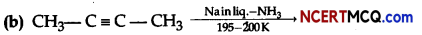(i) Addition of HBr to unsymmetrical alkenes like propene in the presence of light or peroxide takes place following the Markovnikov’s rule. This happens only with HBr but not with HO and HI. Addition of HBr to propene in the presence of benzoyl peroxide follows anti-Markovnikovs rule or peroxide effect or Kharash effect.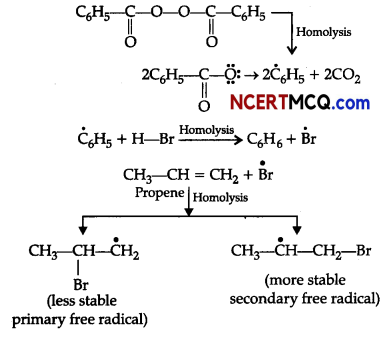Secondary free radicals are more stable than primary radicals. Thus, 1-bromopropane is obtained as the major product.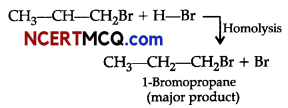(ii) Dehydrohalogenation takes place.OR
(i) (a) 2-methyl 1-butene
(b) 3-methyl- butane-2-one
(ii)
(a) Electrophilic addition reaction is followed. By the Markonikov’s rule, 2-bromopropane is formed as major
product.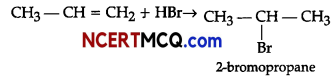(b)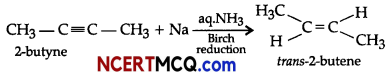Question 10.
An element of Group 2 in the Modem Periodic Table forms covalent oxide which is amphoteric in nature and dissolves in water to give an amphoteric hydroxide. Identify the element and write chemical reactions of the hydroxide of the element with an alkali and an acid.
Since the oxide formed from elements of Group 2 is amphoteric in nature so, the element is beryllium (Be).BeO + H2O → Be(OH)2 Beryllium hydroxide (amphoteric)
Be(OH)2+ 2OH- → [Be(OH)4]2- Beryllate ion
Be(OH)2 + 2HCl + 2H2O → [Be(OH)4 ]Cl2 Tebraquabreylliuxn II chloride

Question 11.
(i) Why alkaline earth metals cannot be obtained by reduction of their oxides?
(ii) Why do beryllium and magnesium not impart colour to the flame in the flame test?
(iii) Among the alkali metals which elements are the most electropositive in nature and lowest in ionic mobility?
OR
On the basis of the figure given below, answer the following questions: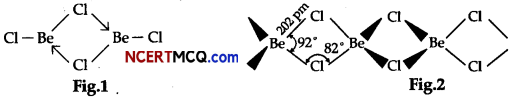(i) What is the difference between the two structures?
(ii) What would be the physical state of BeCl2 in figure 1 and 2?
(iii) Why does Be not exhibit a coordination number more than four?
(i) The alkaline earth metals cannot be obtained by reduction of their oxides because the enthalpies of formation of these oxides are quite high and consequently they are very stable to heat.

(ii) Beryffium and magnesium do not impart colour to the flame in the flame test due to the small size of Be and Mg atoms. So, these atoms require high excitation energy and are not excited by the energy of the flame.

(iii) Cesium is the most electropositive alkali metal because the attraction of nucleus of cesium is very less towards the outermost electron, so it is likely to lose its electron, easily to attain stable noble gas configuration.

Lithium-ion has the smallest size ion due to more effective nuclear charge. Thus, lithium-ion is heavily hydrated that rest element ions of the Group 1. So, Li + has lowest mobility.

OR
(i) Fig 1 is a bridged structure.
Fig 2 is a chain structure

(ii) Structure of BeCl2 in the vapour state:
BeCl2 has bridged chloride structure in the vapour phase.Structure of BeCl2 in the solid-state:
Beryllium chloride has a chain structure in the solid-state.(iii) Beryllium is not readily attacked by acids because of the presence of an oxide film on the surface of the beryllium metal.Section – C

Question 12.
Boyle’s law can be used to describe the effects of altitude on gases in closed cavities within the body, and to calculate the total intra-thoracic gas volume by body plethysmography. As altitude increases, ambient pressure decreases, and therefore, by Boyle’s law, volume expansion occurs in enclosed spaces. This effect can be demonstrated by observing the expansion of a sealed bag of potato chips on an ascending commercial flight.

In one artificial pneumothorax model, a 40 mL pneumothorax increased in volume by up to 16% at 1.5 km (approx. 5000 feet) from sea level, an effect which may prompt thoracostomy before helicopter transfer to prevent transition to a tension pneumothorax. It is estimated that an expansion of up to 30% for a closed volume of gas in the human body, e.g., a bulla, can be expected after ascending from sea level to an altitude of 2.5 km (approx. 8200 feet).

Boyle’s law also explains the use of saline in the cuff of an endotracheal tube during hyperbaric therapy;
to prevent an air leak due to the reduction of volume as pressure increases. When ascending from, depth, if .a diver holds their breath, the gases in their lungs will expand and can cause barotrauma, arterial gas embolism, mediastinal emphysema, or even pneumothorax.
(i) How will you explain the expansion of a sealed packet of chips on a flight which is taking off?
(ii) When the graph of pressure is plotted against volume at different temperatures, a curve is obtained. Identify the curve that corresponds to the constant temperature.
(iii) What does the higher curves in the above-mentioned graph correspond to? Explain with graphical representation?
(iv) Density of a gas is found to be 5.46 g/dm3 at 27°C, 2 bar pressure. What will be its density at STP condition?
OR
34.05 mL of phosphorus vapours weigh 0.0625 g at 546°C and 0.1 bar pressure. What is the molar mass of phosphorus?
(i) According to Boyle’s Law, PV= constant As the flight takes off, altitude increases and the ambient pressure decreases resulting in expansion of volume in enclosed space of the chips packet.

(ii) P – V graph at different values of constant temperature are hyperbolic curves. The curves which correspond to constant temperature are called isotherms.

(iii) According to Boyle’s law
PV =k1Higher curves correspond to higher temperatures. According to P – V diagram, for each curve value of k1 for each curve is different that vary with temperature only.

(iv) Given,
d1 = 5.46 g/dm3
d2= ?
T1 = (27+273)K = 300 K
At STP T2 = (0+273)K = 273K
P1 = 2 bar
At STP P2 = 1 barFrom Ideal gas equation,
d = $$\frac{\mathrm{PM}}{\mathrm{RT}}$$
⇒ $$\frac{d_{1} \mathrm{~T}_{1}}{\mathrm{P}_{1}}=\frac{d_{2} \mathrm{~T}_{2}}{\mathrm{P}_{2}}$$
⇒ $$\frac{5.46 \times 300}{2}=\frac{d_{2} \times 273}{1}$$
⇒ d2 = $$\frac{5.46 \times 300}{273 \times 2}$$ = g/dm3
Hence, density at STP condition is 3g/dm3.
OR
(ii) Given,
P =0.1 bar
V = 34.05 ml=O.03405 L
R0.0821 Latm K-1 mol-1
T = 546+273=819K
From ideal gas,
PV=nRT
n = $$\frac{\mathrm{PV}}{\mathrm{RT}}=\frac{0.1 \times 0.03405}{0.0821 \times 819}$$
=0.00005 mol = 5 x 10-5 mol
∴ Molar mass of phosphorus = $$\frac{\text { Given mass }}{\text { No.of moles }}$$
= $$\frac{0.0625}{0.00005}$$
= 1250 g/mol